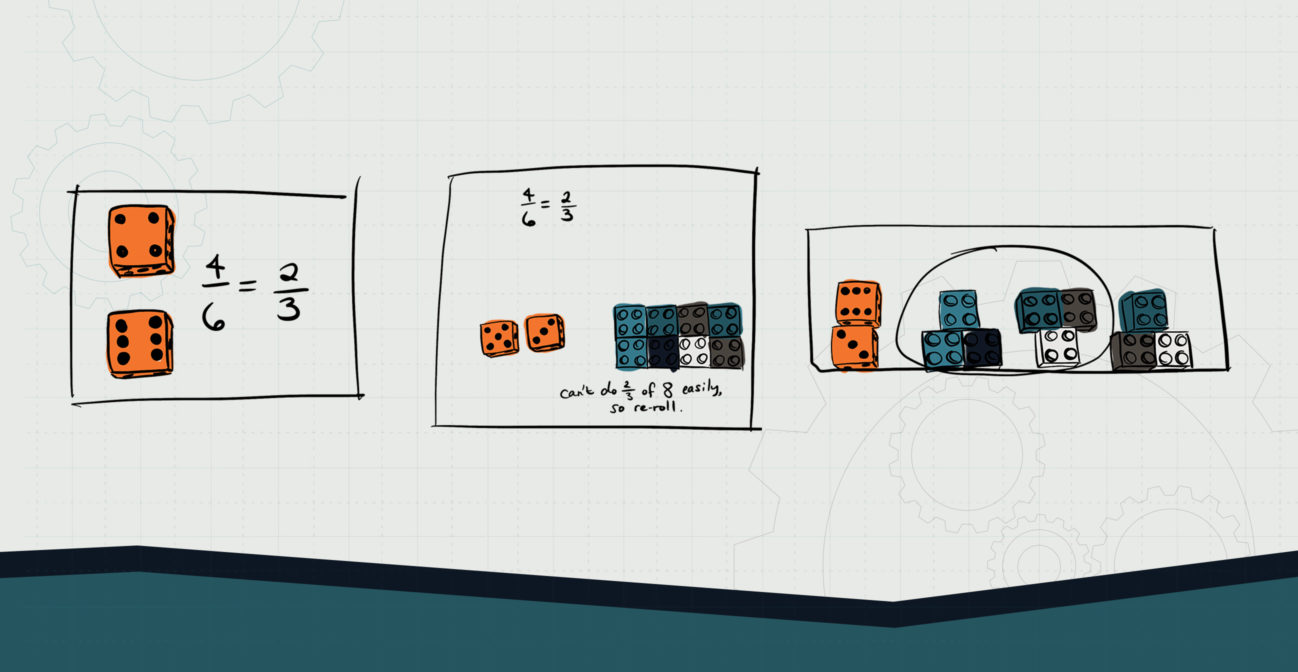# A Roll of the Brick

## Complete fraction multiplication with the assistance of bricks.Objective:
Child will be able to use bricks for beginning fraction multiplication.

Essential Question(s):
When multiplying whole numbers by a fraction, how can we visualize the mathematics?

Special Materials:
Dice, pencil and paper

Bricks Required:
1×2 or 2×2 bricks, 16×16 plates

Project Structure

Engage/Explain:

Explore:

1. Give child a handful of bricks, a 16×16 plate, and a pair of dice.
2. Child rolls the dice to make a fraction, with smaller number on top, then notes it on their paper. If the fraction needs reducing, child should do so.
3. Child rolls the dice again, adds the dice together to determine the whole number, and counts out that many bricks.
4. Ask child to multiply their fraction by the whole number, using the bricks to create groups according to the denominator of the fraction (e.g., if the fraction is ⅘, child divides the bricks into five equal groups). If the bricks can’t be divided evenly by the denominator, the dice should be rolled again to get a new multiplicand.